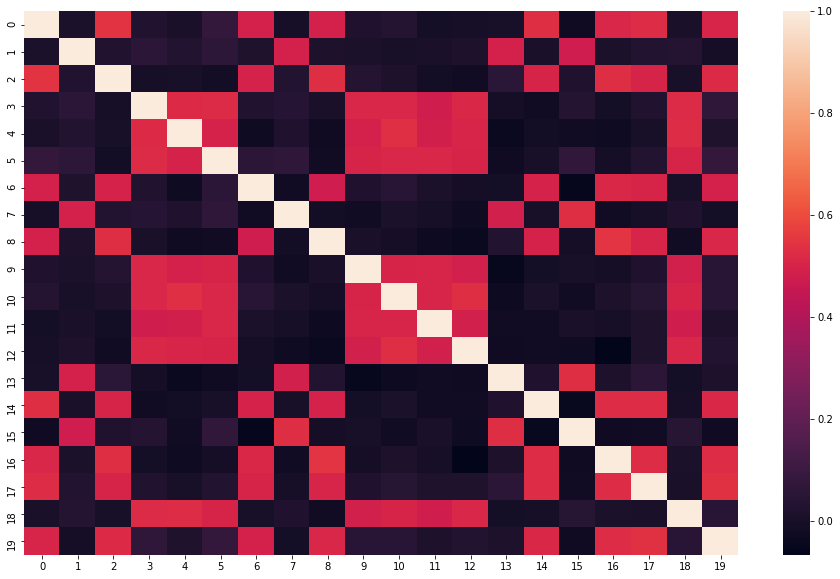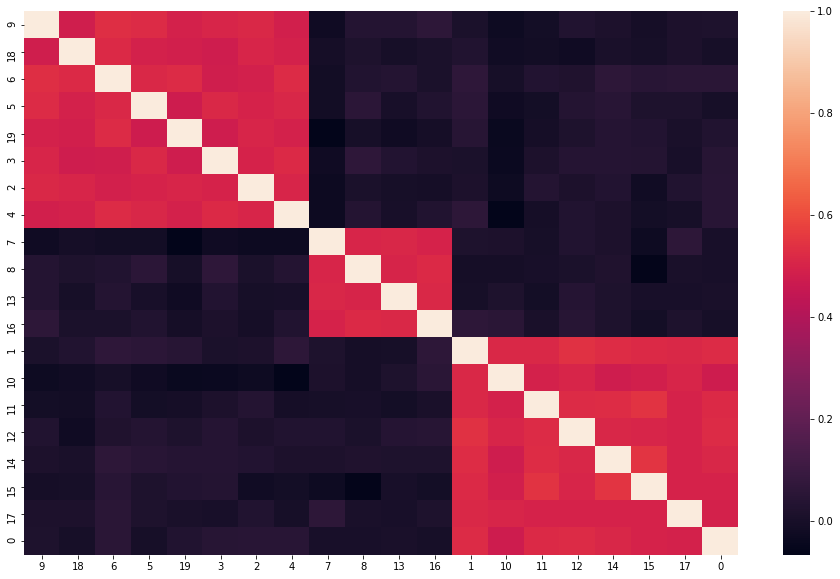# Cluster a Correlation Matrix (in python)

Below is a function to rearrange variables in a correlation matrix (either pandas.DataFrame or numpy.ndarray) to group highly correlated variables near each other.

It turns a correlation matrix that looks like:Into one that looks like:import scipy
import scipy.cluster.hierarchy as sch

def cluster_corr(corr_array, inplace=False):
"""
Rearranges the correlation matrix, corr_array, so that groups of highly
correlated variables are next to eachother

Parameters
----------
corr_array : pandas.DataFrame or numpy.ndarray
a NxN correlation matrix

Returns
-------
pandas.DataFrame or numpy.ndarray
a NxN correlation matrix with the columns and rows rearranged
"""
pairwise_distances = sch.distance.pdist(corr_array)
cluster_distance_threshold = pairwise_distances.max()/2
criterion='distance')
idx = np.argsort(idx_to_cluster_array)

if not inplace:
corr_array = corr_array.copy()

if isinstance(corr_array, pd.DataFrame):
return corr_array.iloc[idx, :].T.iloc[idx, :]
return corr_array[idx, :][:, idx]


You will typically use this function right before calling seaborn.heatmap, as in

#sns.heatmap(df.corr()) # unclustered version
sns.heatmap(cluster_corr(df.corr()))

Please send any thoughts, questions and feedback to wyegelwel at gmail.com or on twitter @wyegelwel